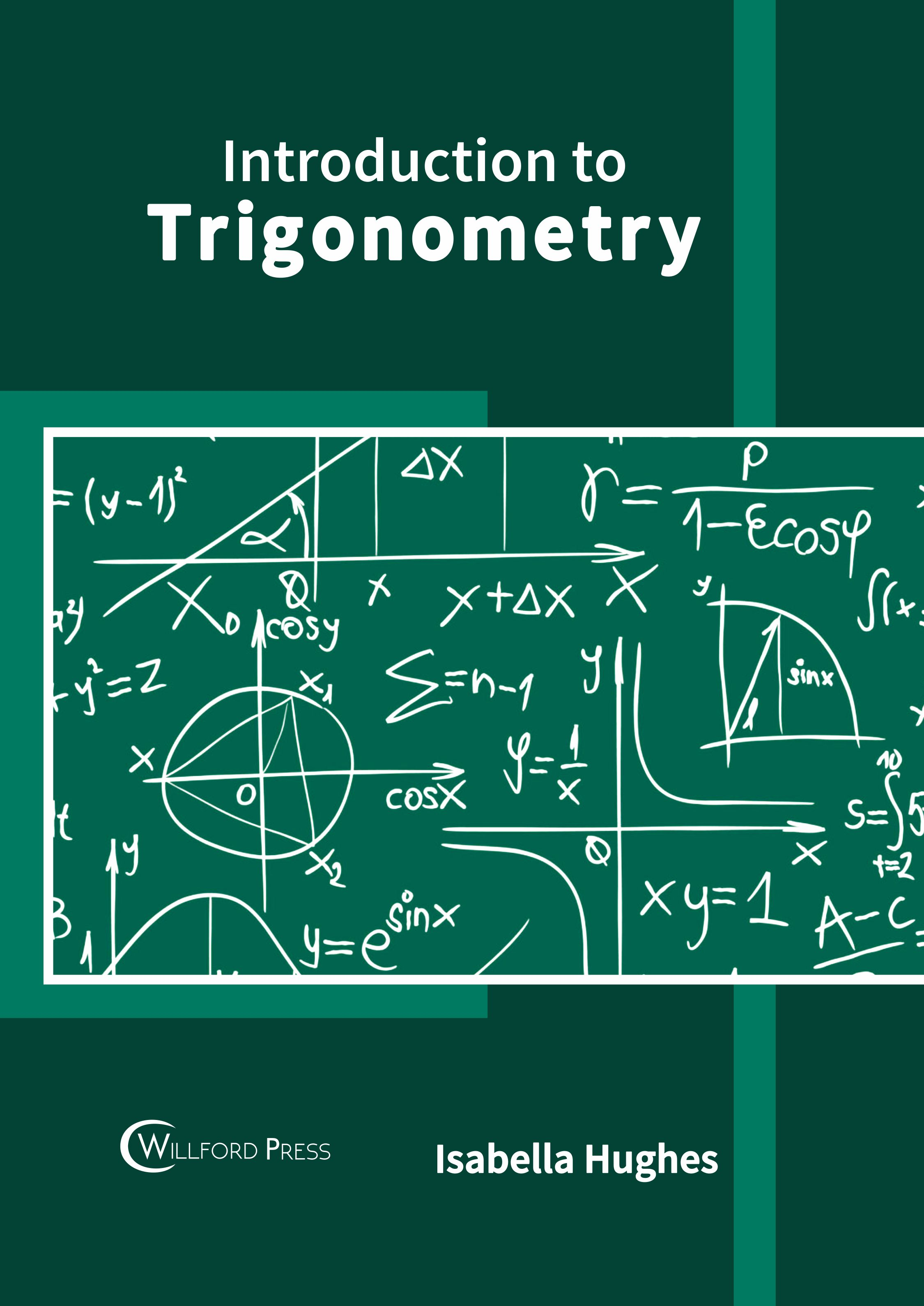BROWSE BY

#### Author

Results 1 - 2 of 2Introduction to Trigonometry Author : Isabella Hughes Subject : Mathematics The branch of mathematics which studies the relationship between side lengths and angles of triangles is known as trigonometry. This field of study is considered to be the foundation of all applied geometry. Read MoreIntroduction to Trigonometry Author : Isabella Hughes Subject : Mathematics The branch of mathematics which studies the relationship between side lengths and angles of triangles is known as trigonometry. This field of study is considered to be the foundation of all applied geometry. Read More
Results 1 - 2 of 2